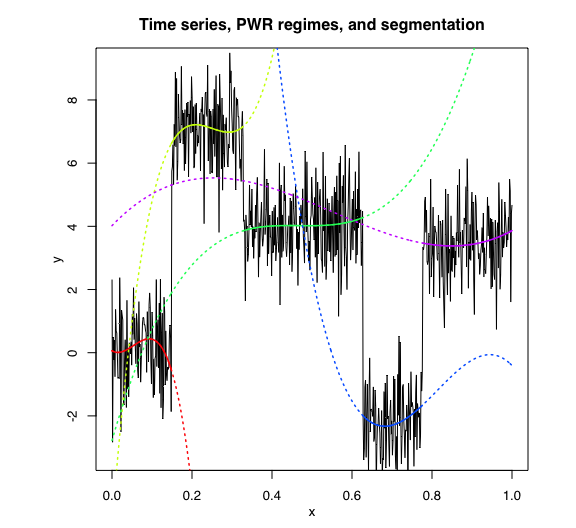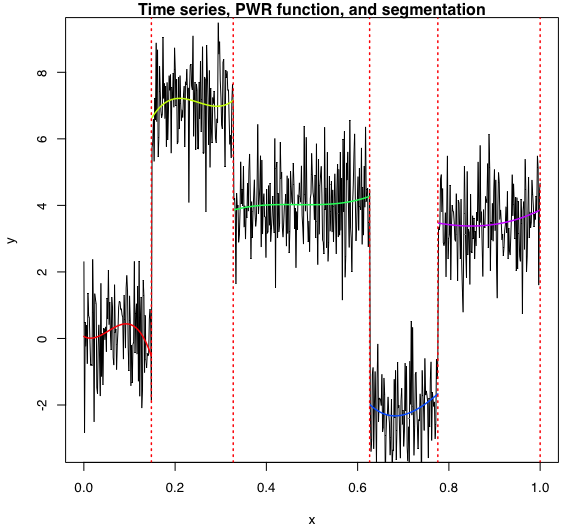Introduction

PWR: Piecewise Regression (PWR) for time series (or structured longitudinal data) modeling and optimal segmentation by using dynamic programming.

It was written in R Markdown, using the knitr package for production.

See help(package="samurais") for further details and references provided by citation("samurais").

data("univtoydataset")

Set up PWR model parameters

K <- 5 # Number of segments
p <- 3 # Polynomial degree

Estimation

pwr <- fitPWRFisher(univtoydataset$x, univtoydataset$y, K, p)

Summary

pwr$summary() ## -------------------- ## Fitted PWR model ## -------------------- ## ## PWR model with K = 5 components: ## ## Clustering table (Number of observations in each regimes): ## ## 1 2 3 4 5 ## 100 120 200 100 150 ## ## Regression coefficients: ## ## Beta(K = 1) Beta(K = 2) Beta(K = 3) Beta(K = 4) Beta(K = 5) ## 1 6.106872e-02 -5.450955 -2.776275 122.7045 4.020809 ## X^1 -7.486945e+00 158.922010 43.915969 -482.8929 13.217587 ## X^2 2.942201e+02 -651.540876 -94.269414 609.6493 -33.787416 ## X^3 -1.828308e+03 866.675017 67.247141 -249.8667 20.412380 ## ## Variances: ## ## Sigma2(K = 1) Sigma2(K = 2) Sigma2(K = 3) Sigma2(K = 4) Sigma2(K = 5) ## 1.220624 1.110193 1.079366 0.9779733 1.028329 Plots Regressors pwr$plot(what = "regressors")Segmentation

pwr\$plot(what = "segmentation")